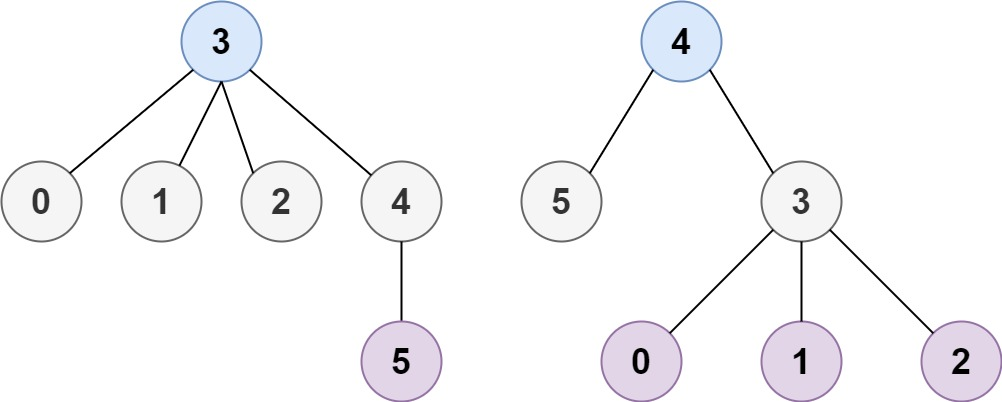## Algorithm

Problem Name: 310. Minimum Height Trees

A tree is an undirected graph in which any two vertices are connected by exactly one path. In other words, any connected graph without simple cycles is a tree.

Given a tree of `n` nodes labelled from `0` to `n - 1`, and an array of `n - 1` `edges` where `edges[i] = [ai, bi]` indicates that there is an undirected edge between the two nodes `ai` and `bi` in the tree, you can choose any node of the tree as the root. When you select a node `x` as the root, the result tree has height `h`. Among all possible rooted trees, those with minimum height (i.e. `min(h)`)  are called minimum height trees (MHTs).

Return a list of all MHTs' root labels. You can return the answer in any order.

The height of a rooted tree is the number of edges on the longest downward path between the root and a leaf.

Example 1:```Input: n = 4, edges = [[1,0],[1,2],[1,3]]
Output: 
Explanation: As shown, the height of the tree is 1 when the root is the node with label 1 which is the only MHT.
```

Example 2:```Input: n = 6, edges = [[3,0],[3,1],[3,2],[3,4],[5,4]]
Output: [3,4]
```

Constraints:

• `1 <= n <= 2 * 104`
• `edges.length == n - 1`
• `0 <= ai, bi < n`
• `ai != bi`
• All the pairs `(ai, bi)` are distinct.
• The given input is guaranteed to be a tree and there will be no repeated edges.

## Code Examples

### #1 Code Example with C++ Programming

```Code - C++ Programming```

``````
class Solution {
public:
vector findMinHeightTrees(int n, vector>& edges) {
vectorres;
vector>graph(n);
// Build Graph
for(auto x: edges){
graph[x.first].push_back(x.second);
graph[x.second].push_back(x.first);
}
int minHeight = INT_MAX;
// BFS
for(int i = 0; i < n; i++){
if(graph[i].size() < 5 && n > 10000) continue; // Magic for passing the last TC.
vectorvisited(n);
int height = 0;
dequecur;
dequesub;
cur.push_back(i);

while(!cur.empty() && height <= minHeight){
int node = cur.front();
cur.pop_front();
visited[node] = 1;
for(auto neigh: graph[node])
if(!visited[neigh]) sub.push_back(neigh);
if(cur.empty()){
height++;
swap(cur, sub);
}
}
if(height < minHeight){
res.clear();
minHeight = height;
res.push_back(i);
}
else if(minHeight == height) res.push_back(i);
}
return res;
}
};
``````
Copy The Code &

Input

cmd
n = 4, edges = [[1,0],[1,2],[1,3]]

Output

cmd


### #2 Code Example with Java Programming

```Code - Java Programming```

``````
class Solution {
public List findMinHeightTrees(int n, int[][] edges) {
if (n == 1) {
return Collections.singletonList(0);
}
Map> map = new HashMap<>();
for (int[] edge : edges) {
}
List leaves = new ArrayList<>();
for (int i = 0; i < n; i++) {
if (map.getOrDefault(i, new HashSet<>()).size() == 1) {
}
}
int count = n;
while (count > 2) {
int size = leaves.size();
count -= size;
List newLeaves = new ArrayList<>();
for (int i = 0; i < size; i++) {
int leaf = leaves.get(i);
for (int toRemove : map.getOrDefault(leaf, new HashSet<>())) {
map.get(toRemove).remove(leaf);
if (map.get(toRemove).size() == 1) {
}
}
}
leaves = newLeaves;
}
return leaves;
}
}
``````
Copy The Code &

Input

cmd
n = 4, edges = [[1,0],[1,2],[1,3]]

Output

cmd


### #3 Code Example with Javascript Programming

```Code - Javascript Programming```

``````
const findMinHeightTrees = function (n, edges) {
const graph = {}

for(const [u, v] of edges) {
if(graph[u] == null) graph[u] = new Set()
if(graph[v] == null) graph[v] = new Set()
}

let q = []
for(let i = 0; i < n; i++) {
if(graph[i].size === 1) q.push(i)
}

while(n > 2) {
const size = q.length, nxt = []
n -= size
for(let i = 0; i < size; i++) {
const cur = q[i]
for(const e of (graph[cur] || [])) {
graph[e].delete(cur)
if(graph[e].size === 1) nxt.push(e)
}
}

q = nxt
}

return q
}
``````
Copy The Code &

Input

cmd
n = 6, edges = [[3,0],[3,1],[3,2],[3,4],[5,4]]

Output

cmd
[3,4]

### #4 Code Example with Python Programming

```Code - Python Programming```

``````
class Solution:
def findMinHeightTrees(self, n, edges):
if n == 1: return 
adj = [set() for i in range(n)]
for i, j in edges:
leaves = [i for i in range(n) if len(adj[i]) == 1]
while n > 2:
n -= len(leaves)
newleaves = []
for i in leaves:
newleaves.append(j)
leaves = newleaves
return leaves
``````
Copy The Code &

Input

cmd
n = 6, edges = [[3,0],[3,1],[3,2],[3,4],[5,4]]

Output

cmd
[3,4]